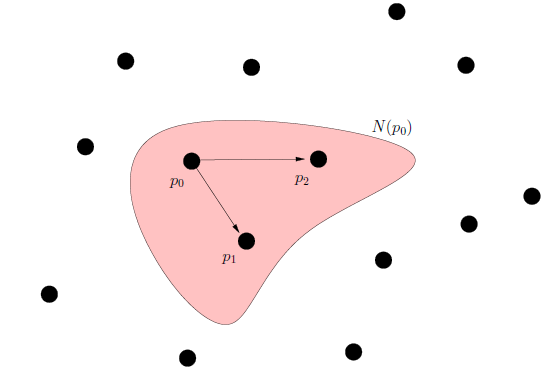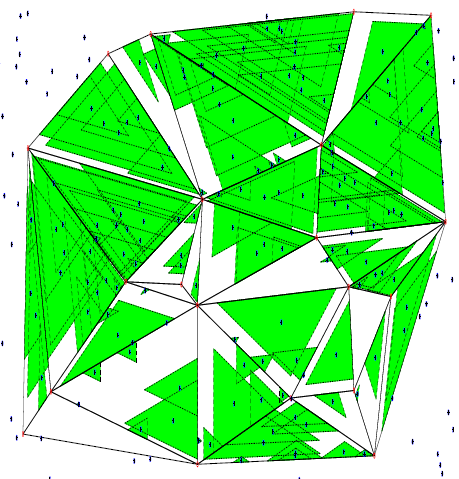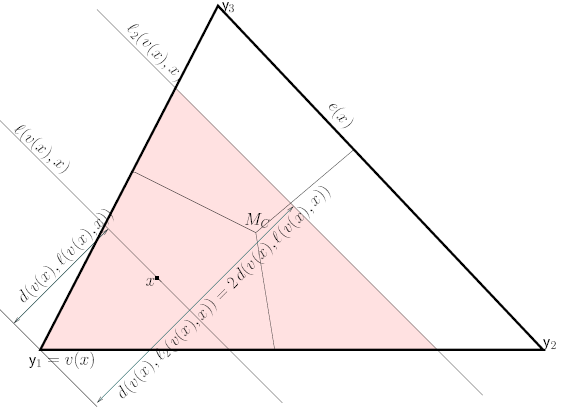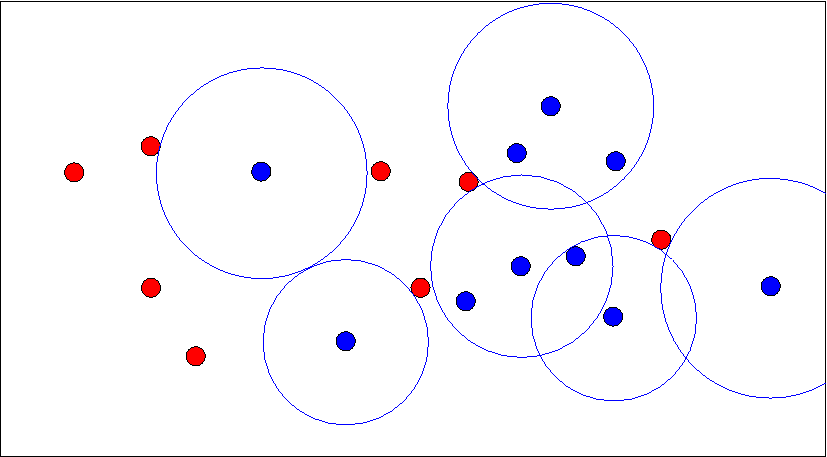• Let $$(\Omega,\mathcal{M})$$ be a measureable space. The proximity map $$N(.)$$ is given by $$N : \Omega \longrightarrow \wp(\Omega)$$, where $$\wp(.)$$ is the power set functional. Hence, proximity region of $$x \in \Omega$$ is denoted by $$N(x)$$.
• A point $$p \in N(x)$$ is thought of as being "closer" to $$x \in \Omega$$ than the points in $$\Omega \setminus N(x)$$.
• The proximity catch digraph $$D=D(\mathcal{V},\mathcal{A})$$ has a vertex set $$\mathcal{V}=\{y_1,\cdots,y_n\}$$ and the arc set $$\mathcal{A}$$ is defined by $$(v_i,v_j) \in \mathcal{A}$$ if and only if $$v_j \in N(v_i)$$ for $$i \neq j$$.
•